## DEV Community 👩‍💻👨‍💻

Eugenia

Posted on • Originally published at Medium on

# Visualizing Twitter interactions with NetworkX#### Connections, connections, and connections…

Social media is used every day for many purposes: expressing opinions about different topics such as products and movies, advertising an event, a service or a conference, among other things. But what is most interesting about social media, and particularly for this post, about Twitter, is that it creates connections; networks that can be studied to understand how people interact or how news and opinions get spread.

Previously, we have used Twitter API to store tweets to afterward performed a sentiment analysis and elucidate the public opinion about avengers. Let’s review the steps needed to stream tweets: First of all, we should go to the Twitter developer website, log-in with the twitter account and ask for approval as a developer. After receiving the approval, we go on and create a new app filling out the details, and lastly, we create the access tokens keeping them in a safe place. In a Jupyter notebook, we can use the Tweepy Python library to connect with our Twitter credentials and stream real-time tweets related to a term of interest and then, save them into a .txt file.

Now, we are going to read all the data we gathered into a pandas DataFrame.

We will use this information to graph how the people that tweet about Avengers interact with each other. There are three types of interactions between two Twitter users that we are interested in: retweets, replies, and mentions. According to Twitter documentation, the JSON file retrieved representing the Tweet object will include a User object that describes the author of the Tweet, an entities object that includes arrays of hashtags and user mentions, among others.

Let’s take a look at the columns of our DataFrame so we can check how the information was read: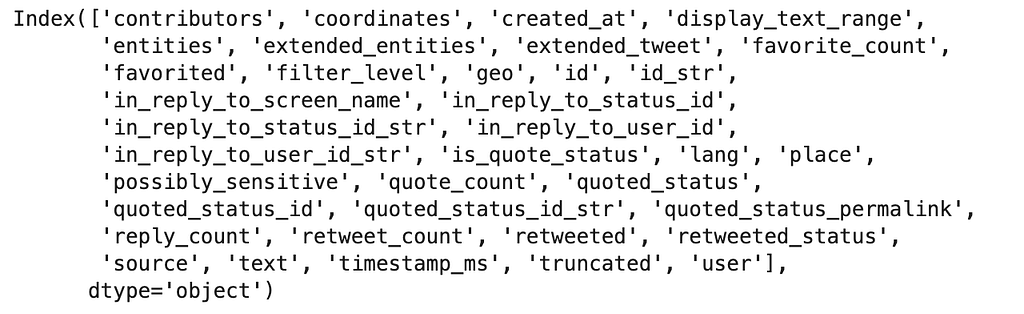From the displayed columns, we are interested in:

• Author of the Tweet: Name(screen_name) and Id(id ) inside user.
• Twitter users mention in the text of the Tweet: Name and Id can be found as screen_name and id in user_mentions inside entities.
• Account taking the retweet action: screen_name and id inside user object of the retweet_status.
• Tweet to which the tweet replies to: in_reply_to_status_id

You may be wondering how the details collected will help us build a network representing the interactions between Twitter users. In order to answer that, we will need to take a glimpse at Graph Data Structure.

Let’s start with the basic concepts. What is a Graph? Graph is a data structure used to represent and analyze connections between elements. Its two main elements are nodes or vertices, and edges or lines that connect two nodes.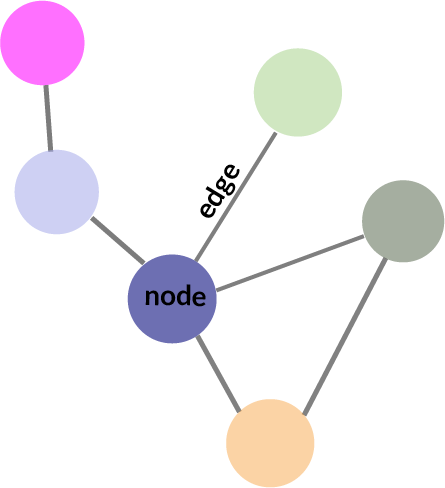Even though two nodes could not be directly connected to each other, there is a sequence of edges or path that can be followed to go from one node to the other. The possibility of finding one node by following paths is what makes Graph so powerful to represent different structures or networks. When there are a few nodes such that there is no path you can take to reach them, then we are in the presence of a disconnected graph or isolated nodes.

Graph can also be classified as directed when the edges have a specific orientation (normally representing by an arrow to indicate direction) or undirected when the edges don’t follow any orientation.

In our analysis, users represent the nodes of our Graph or Network. If we find any type of interaction (retweet, reply or mention) between them, an edge will be created to connect both nodes. We can work with direct Graph if we are interested in knowing which user retweets another user. Because we only want to describe the interaction present between two users without caring about its orientation, then we are going to use an Undirected Graph.

The next question is which tool can be used in our analysis. We will take advantage of NetworkX, a Python package for the creation and study of the structure of complex networks, such as a social network.

First of all, we’ll define a function that will allow us to get a list of the interactions between users. We will iterate over the DataFrame, obtain the user_id and screen_name of the user that the author of that specific tweet mention, reply or retweet. The function will return the user of the specific tweet together with a list of the users with whom the user interacted. We need to be careful to discard any None value that may be raised if the user doesn't have any interactions in the three categories mentioned before.

Now, it’s time to initialize the Graph. We can do this by calling the function .Graph() of NetworkX.

There are two other important functions to create a Graph. The first one is add_node()and the second one, add_edge both with a very descriptive name. Let’s pay attention to the syntax of add_edge:

Graph.add_edge(u_of_edge **,** v_of_edge** , **** **attr***)*

where u, v are the nodes, and attr are keyword arguments that characterize the edge data such as weight, capacity, length, etc.

If we add an edge that already exists, the edge data will get updated. Also, if we are an edge between two nodes that are still not in the Graph, the nodes will be created in the process.

We are going to populate the Graph by calling the function get_interactions that we defined earlier. With this information, we apply the function add_edge to every tuple consisting of the tweet’s user_id and the user_id of the user mentioned, replied to or retweeted, creating the nodes and the edges connecting them. Also, the tweet id will be added as edge data.

Now that we have the node and edge of the Graph created, let’s see the number of nodes and edges present:Let’s explore now some other characteristic of a Graph. The degree of a node u, denoted as deg(u), is the number of edges that occur to that node. In simpler words, the number of connections a particular node has. The maximum degree of a graph and the minimum degree of a graph are the maximum and minimum degree of its nodes, respectively.

In our case, we can obtain the degrees of the Graph:

and the maximum and minimum degree: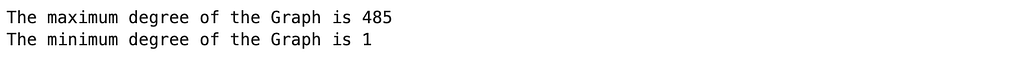We can also obtain the average degree and the most frequent degree of the nodes in the Graph:An undirected graph is connected if, for every pair of nodes, there is a path between them. For that to happen, most of the nodes should have at least a degree of two, except for those denominated leaves which have a degree of 1. From the characteristics of the Graph, we can suspect that the graph is not connected. In order to confirm these, we can use nx.is_connected: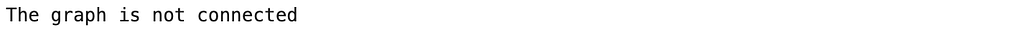Components of a graph are the distinct maximally connected subgraphs. Now, that we confirm that our Graph is not connected, we can check how many connected components it has: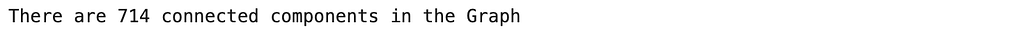For this analysis, we are going to work with the largest connected component. Fortunately, NetworkX gives us an easy way to obtain that component by using nx.connected_component_subgraphs that generates graphs, one for each connected component of our original graph, and max function that will help us retrieve the largest component:

Now, we can obtain the characteristics of this new graph: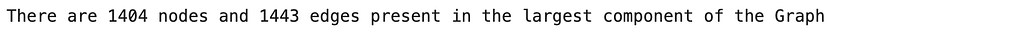And if we use the function nx.is_connected we’ll observe that the subgraph is connected as it should be.

Clustering and transitivity measure the tendency for nodes to cluster together or for edges to form triangles. In our context, they are measures of the extent to which the users interacting with one particular user tend to interact with each other as well. The difference is that transitivity weights nodes with a large degree higher.

The clustering coefficient, a measure of the number of triangles in a graph, is calculated as the number of triangles connected to node i divided by the number of sets of two edges connected to node i (Triple nodes). While the transitivity coefficient is calculated as 3 multiply by the number of triangles in the network divided by the number of connected triples of nodes in the network. These two parameters are very important when analyzing social networks because it gives us an insight into how users tend to create tightly knot groups characterized by relatively high-dense ties.

Let’s take a look at what is happening in our analysis using the functions average_clustering and transitivity: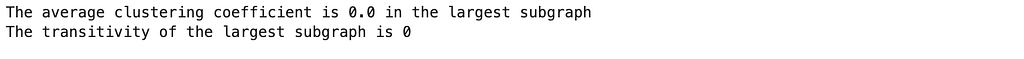It appears that in our graph, the users do not tend to form close clusterings.

After that, we’ll investigate some summary statistics, particularly related to distance, or how far away one node is from another random node. Diameter represents the maximum distance between any pair of nodes while the average distance tells us the average distance between any two nodes in the network. NetworkX facilitates the functions diameter and average_shortest_path_length to obtain these parameters:Now, we are going to focus on network centrality which captures the importance of a node’s position in the network considering: degree on the assumption that an important node will have many connections_, closeness_ on the assumption that important nodes are close to other nodes_, and finally, betweenness_ on the assumption that important nodes are well situated and connect other nodes. For this, we are going to use the following functions degree_centrality, closenness_centrality and betwenness_centrality, all which return a list of each node and its centrality score. We will particularly capture the node with the best score in each one.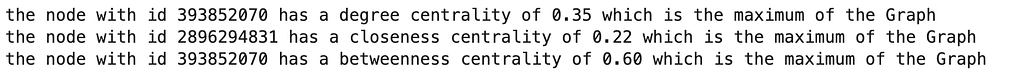As we can see not always the same node shows the maximum in all the centrality measures. However, the node with id 393852070 seems to be the one with more connection that is well situated connecting other nodes. On the other hand, the node with id 2896294831 is closest to other nodes.

Now, we can get to see how the Graph looks like. For that, we will use nx.drawing.layout to apply node positioning algorithms for the graph drawing. Specifically, we will use spring_layout that uses force-directed graph drawing which purpose is to position the nodes in two-dimensional space so that all the edges are of equal length and there are as few crossing edges as possible. It achieves this by assigning forces among the set of edges and nodes based on their relative positions and then uses this to simulate the motion of the edges and nodes. One of the parameters that we can adjust is k, the optimal distance between nodes; as we increase the value, the nodes will farther apart. Once, that we got the positions, we are also going to create a special list so that we can draw the two nodes with higher centrality that we found in different colors to highlight them.

After all that calculation, we’ll use the functions draw_networkx_nodes() and draw().

And finally, we have the drawing of the largest connected component of our original Graph: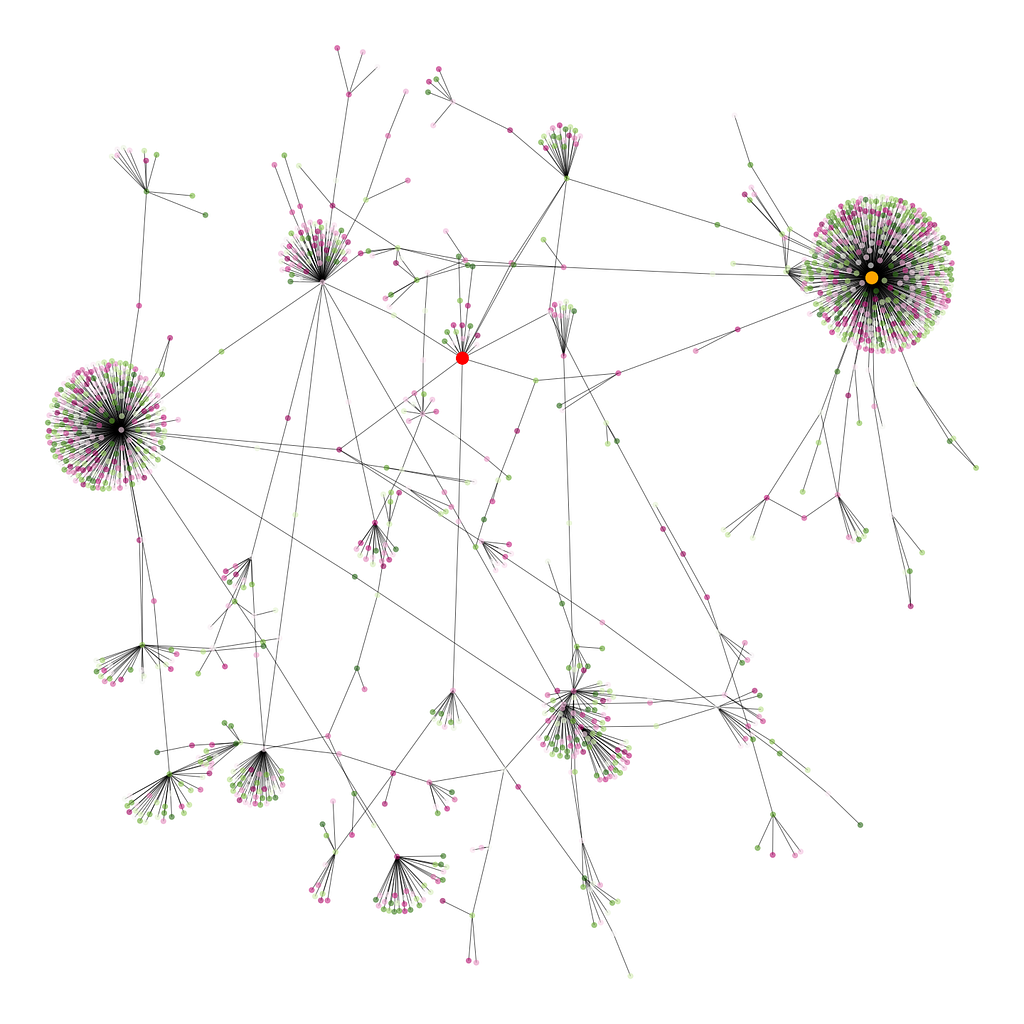If you want to get to know the entire code for this project, check out my GitHub Repository.Arne • Edited on

Good content! I am working on a SNA project at the moment and I am applying some of what you wrote to it. I’m surprised how little is being written about this topic... Thanks for sharing!Andy Piper

This is very cool, thank you for sharing!

## Every Week

We have a Welcome Thread where we invite members to tell us a bit about themselves. Join the conversation with us!